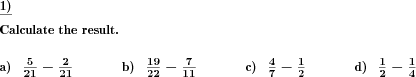Custom math worksheets at your fingertips# Details for problem "Fractions addition subtraction"

Quickname: 6408

Elementary School, Primary School, Junior High School, Middle School, High School.

## Example## Description

A number of fractions has to be added or subtracted in a consecutive way. The number of problems and the number of fractions for each problem is selectable.

The fractions may be unlike quantities, in which case they have to be converted to like quantities first. The problem may be set to produce only chains of like quantities - or only unlike quantities.

The numerator and denominator of each fraction is taken from a preset number range.

The fractions' sign may be chosen to be positive (for addition) only, negative (for subtraction) only, or chosen randomly.

Improper fractions can be allowed, disallowed or enforced. In case they may occur, it can be chosen whether they will be output as improper fractions or mixed numbers.

Download free printable worksheets for this math problem here. The worksheet contains the problems only, the solution sheet includes the answers. Just click on the respective link.

•Worksheet 1Solution sheet with answers
•Worksheet 2Solution sheet with answers
•Worksheet 3Solution sheet with answers

If you can not see the solution sheets for download, they may be filtered out by an ad blocker that you may have installed. If this is the case, please allow ads for this page and reload the page. The solution sheets will then reappear.

• Do these sample worksheets do not really fit?
• Do you need more math worksheets, with a different level of difficulty?
• Would you like to combine different problems on a worksheet and adjust them to your needs?
• As a teacher, you can put together your own worksheets using the automatically generated math problems provided.
With a free initial credit, you can start creating your own math worksheets in a few minutes.

You can try it for free! Register here, to create custom worksheets now!

## Customization options for this problem

Parameter
Possible values
Number of problems
1, 2, 3, 4, 5, 6, 7, 8, 9, 10
Plus/Minus
Mixed, Plus, Minus
Number range
20, 30, 40, 50, 100, 200, 500, 1000, 10000, 100000
Number of fractions
1, 2, 3, 4
Improper fractions
No, Yes, as a fraction, Yes, as a mixed number
All like quantities
Never, May, Always

## Similar problems

Remark
Description
Like quantities are required for the addition and subtraction of fractions.
Convert multiple fractions to like quantities
With the result given, a summand is blanked out and to be filled in correctly
In a sum term of fractions, blanks have to be filled in correctlyDeutsche Version dieser Aufgabe
These informational pages with samples describe math problems that can be combined on custom math worksheets with solutions for home and K-12 school use.
Deutsche Seiten
×